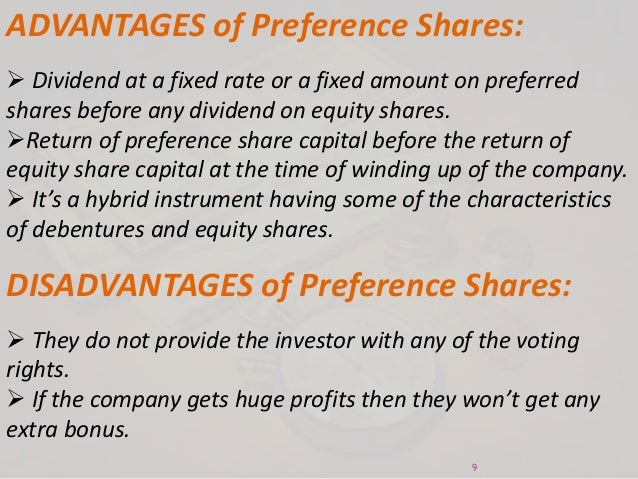# Valuation of Preference Shares

Phone

#### Present Value of Perpetual or an Irredeemable Preference Share

##### The present value of irredeemable preference share can be determined by simply discounting the streams of dividend payment for the infinite period by an appropriate capitalization rate of the discount rate. Formula: V = D/Kp Where, V = Present value of the preference share. D = Annual dividend payment on the preference share. Kp = Capitalization rate.